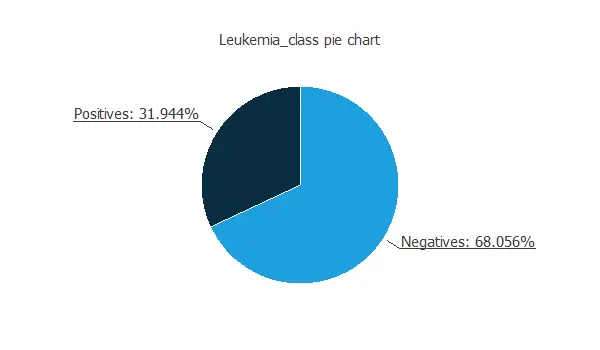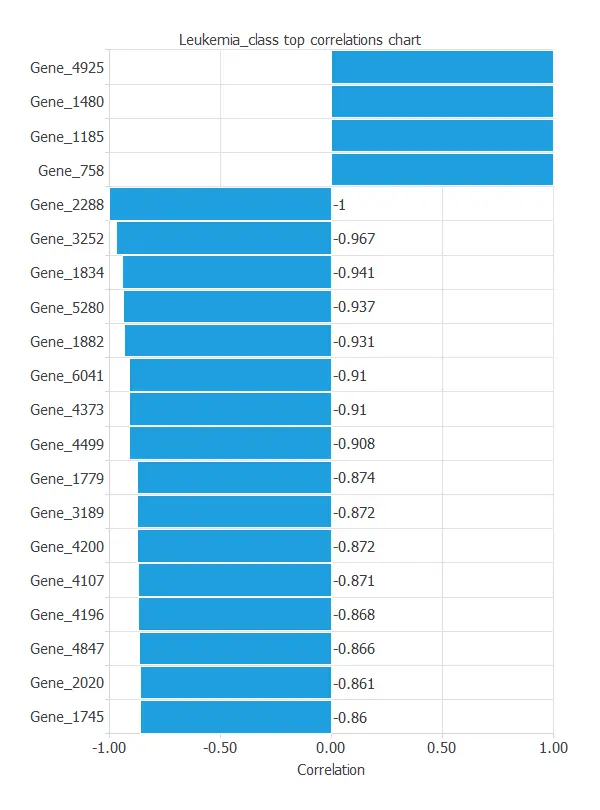﻿ Leukemia diagnosis from microarray data using machine learning

# Diagnose the type of leukemia from microarray data using artificial intelligence

The purpose of this example is to diagnose the leukemia of patients, acute lymphoblastic leukemia (ALL) or acute myeloid leukemia (AML), depending on their DNA coding.

Furthermore, because of the large DNA code, a gene selection will be performed to simplify the model and better understand the disease.

The DNA is coded in 7129 genes; each takes a value between 0 and 1. The model's output is a binary value; it takes the value 0 for acute lymphoblastic leukemia (ALL) and the value 1 for acute myeloid leukemia (AML).### Contents:

This example is solved with Neural Designer. To follow it step by step, you can use the free trial.

## 1. Application type

The variable to be predicted is binary (ALL or AML). Thus, this is a classification project

The goal here is to model ALL probability, conditioned on the microarray signals. Note that the probability of AML is 1 - ALL.

## 2. Data set

The data file contains a total of 7129 genes and 72 patients. The first row in the data file contains the names of the variables, and the rest represent the instances.

• Gene 1
• ···
• Gene 7129
• 0 acute lymphoblastic leukemia (ALL) and the value 1 for the acute myeloid leukemia (AML).

The data distribution tells us the percentages of ALL and AML for the current dataset.The inputs-targets correlations indicate to us which genes are more related to ALL or AML diseases.As we can see in the previous figure, some genes have a high correlation with the diagnosis. The gene 4847 and the gene 2288 perfectly correlate with the target variable.

This correlation means that these genes greatly impact the target variable. To do that, their values must be logistically separable. A certain probability is attached to the random logistical separability of a column with 72 values. It obeys the formula:n1 and n2 being the total number of values of AML and ALL respectively.

This would mean that, for a dataset similar to ours, but with its values set randomly, there may be 1.831·10-15 of the variables that are very correlated only by chance and not by actual correlation. In this case, that number is very small and doesn't affect our conclusions at all.

## 3. Neural network

The second step is to choose a neural network to represent the classification function. For classification problems, it is composed of:

However, due to the massive number of variables in this dataset, we do not define the neural network yet.

## 4. Training strategy

The training strategy is applied to the neural network to obtain the best possible performance. It is composed of two things:

• A loss index.
• An optimization algorithm.

We will not perform the training strategy for this example. As previously stated, the dataset contains a large number of variables. Therefore, we will perform a proper model selection before making choices about the neural network or the training strategy.

## 5. Model selection

Due to the high number of variables in the microarray, a feature selection should be performed. The input selection is used to find the optimal subset of inputs for the model's best performance.

In this example, the input selection algorithm selected is the growing inputs. This method is optimum for this kind of problem.

The next table shows the results of the input selection.

Value
Optimal number of inputs 1
Optimum training error 0.0297108
Optimum selection error 0.0926624
Iterations number 0
Elapsed time 00:00

We can observe that the algorithm did no iterations. This is because it found a variable with perfect correlation, and it stopped with this variable as the only input.

Order selection algorithms train several network architectures with a different number of neurons and select that with the smallest selection error.

The incremental order method starts with a small number of neurons and increases the complexity at each iteration. The following chart shows the training error (blue) and the selection error (orange) as a function of the number of neurons.The final selection error achieved is 0.029 for an optimal number of neurons of 1.

The final neural network is displayed below.## 6. Testing analysis

A standard method for testing the prediction capabilities is to compare the outputs from the neural network against an independent set of data. The correlation matrix shows which instances are misclassified.

Predicted positive Predicted negative
Real positive 4 0
Real negative 0 10

As we can see in this confusion matrix, the model perfectly predicts the leukemia class with independent data to those used for the training and the input selection.

### 7. Model deployment

Once the model is obtained, Neural Designer provides the user with the mathematical expression in several programming languages. The file leukemia.py contains the model in python language.

## References

• Golub,T.R., Slonim,D.K., Tamayo,P., "Molecular Classification of Cancer: Class Discovery and Class Prediction by Gene Expression Monitoring", Science, Vol. 286, pp. 531-537 (1998).

## Related posts:The development of this application has been funded by the NEMHESYS - NGS Establishment in Multidisciplinary Healthcare Education System project.